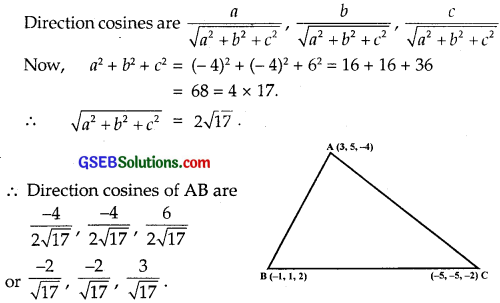# GSEB Solutions Class 12 Maths Chapter 11 Three Dimensional Geometry Ex 11.1

Gujarat Board GSEB Textbook Solutions Class 12 Maths Chapter 11 Three Dimensional Geometry Ex 11.1 Textbook Questions and Answers.

## Gujarat Board Textbook Solutions Class 12 Maths Chapter 11 Three Dimensional Geometry Ex 11.1

Question 1.
If a line makes angles 90°, 135° and 45° with x, y and z axes respectively, find its direction cosines.
Solution:
Direction angles are 90°, 135° and 45°.
∴ Direction cosines are
l = cos 90° = 0, m = cos 135° = – $$\frac{1}{\sqrt{2}}$$ and n = cos 45° = $$\frac{1}{\sqrt{2}}$$.
Hence, direction cosines are 0, – $$\frac{1}{\sqrt{2}}$$, $$\frac{1}{\sqrt{2}}$$.

Online Area Between Two Curves Calculator helps you to evaluate the equations and give the exact area between two curves in a short span of time.Question 2.
Find the direction cosines of a line which makes equal angles with coordinate axes.
Solution:
Let direction angle be α each
∴ Direction cosines are cos α, cos α, cos α.
But l2 + m2 + n2 = 1, where l, m, n are the direction cosines of the line.
∴ cos2α + cos2α + cos2α = 1 .
⇒ 3cos2α = 1
∴ cos α = ± $$\frac{1}{\sqrt{3}}$$.
Thus, the direction cosines, of the line equally inclined to the coordinate axes are ± $$\frac{1}{\sqrt{3}}$$, ± $$\frac{1}{\sqrt{3}}$$, ± $$\frac{1}{\sqrt{3}}$$.Question 3.
If a line has the direction ratios – 18, 12 and – 4, then what are its direction cosines?
Solution:
Direction ratios of a line are – 18, 12 and – 4.
Now, $$\sqrt{(-18)^{2}+(12)^{2}+(-4)^{2}}$$ = $$\sqrt{484}$$ = $$\sqrt{2×2×11×11}$$
= 2 × 11 = 22
∴ Direction cosines are $$\frac{- 18}{22}$$, $$\frac{12}{22}$$, $$\frac{- 4}{22}$$ or $$\frac{- 9}{11}$$, $$\frac{6}{11}$$, $$\frac{- 2}{11}$$.

Question 4.
Show that the points (2, 3, 4), (- 1, – 2, 1) and (5, 8, 7) are collinear.
Solution:
The given points are
A(2, 3, 4), B(- 1, – 2, 1) and C(5, 8, 7).
Direction ratios of AB are x2 – x1, y2 – y1, z2 – z1
i.e; (- 1 – 2), (- 2 – 3), (1 – 4)
or – 3, 5, – 3
Direction ratios of BC are
5 – (- 1), 8 – (- 2), (7 – 1) or 6, 10, 6,
which are – 2 times the direction ratios of AB.
∴ AB and BC have the same direction ratios.
∴ AB || BC. But B is a common point of AB and BC.
Hence, A, B and C are collinear.Question 5.
Find the direction cosines of the sides of the triangle whose vertices are (3, 5, – 4), (- 1, 1, 2) and (- 5, – 5, – 2).
Solution:
The vertices of triangle ABC are
A (3, 5, – 4), B(- 1, 1, 2) and C(- 5, – 5, – 2).

(i) Direction ratios of AB are x2 – x1, y2 – y1, z2 – z1
or – 1 – 3,1 – 5 and 2 – (- 4), i.e., – 4, – 4 and 6.(ii) Points B and C are (- 1, 1, 2) and (- 5, – 5, – 2) respectively.
∴ Direction ratios of BC are
– 5 – (- 1), – 5 – 1, – 2 – 2 or – 4, – 6 – 4
or – 2, – 3, – 2.
∴ $$\sqrt{(a)^{2}+(b)^{2}+(c)^{2}}$$ = $$\sqrt{4+9+4}$$ = $$\sqrt{17}$$.
⇒ Direction cosines of BC are
$$\frac{-2}{\sqrt{17}}$$, $$\frac{-3}{\sqrt{17}}$$, $$\frac{-2}{\sqrt{17}}$$.

(iii) Points C and A are (- 5, – 5, – 2) and (3, 5, – 4) respectively.
∴ Direction ratios of CA are
3 – (- 5), 5 – (- 5), – 4 – (- 2) or 8, 10, – 2 or 4, 5, – 1.
∴ $$\sqrt{(a)^{2}+(b)^{2}+(c)^{2}}$$ = $$\sqrt{(4)^{2}+(5)^{2}+(1)^{2}}$$ = $$\sqrt{16+15+1}$$ = $$\sqrt{42}$$.
∴ Direction cosines of CA are
$$\frac{4}{\sqrt{42}}$$, $$\frac{5}{\sqrt{42}}$$, $$\frac{-1}{\sqrt{42}}$$
Thus, the direction cosines of AB, BC and CA are respectively.
$$\frac{-2}{\sqrt{17}}$$, $$\frac{-2}{\sqrt{17}}$$, $$\frac{3}{\sqrt{17}}$$; $$\frac{-2}{\sqrt{17}}$$, $$\frac{-3}{\sqrt{17}}$$, $$\frac{-2}{\sqrt{17}}$$ and $$\frac{4}{\sqrt{42}}$$, $$\frac{5}{\sqrt{42}}$$, $$\frac{-1}{\sqrt{42}}$$.